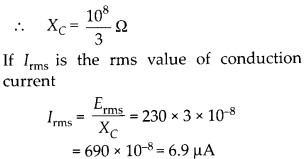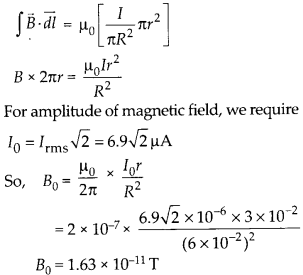Enlightened

# Question 2: NCERT Solutions for 12th Class Physics: Chapter 8-Electromagnetic Waves

• 0

Question 2: NCERT Solutions for 12th Class Physics: Chapter 8-Electromagnetic Waves

A parallel plate capacitor as shown in figure made of circular plates each of radius R = 6.0 cm has a capacitance C = 100 pF. The capacitor is connected to a 230 V ac supply with a (angular) frequency of 300 rad s-1.
(a) What is the rms value of the conduction current?
(b) Is the conduction current equal to the displacement current?
(c) Determine the amplitude of 6 at a point 3.0 cm from the axis between the plates.Share

1. Solution:
(a) Capacity of capacitor C = 100 pF(b) Yes, the conduction current in wires is always equal to displacement current within plates.
(c)To find magnetic field B of a point 3 cm from the axis within plates, let us assume a loop of radius 3 cm with center on axis. Now modified Ampere’s Law.Check the complete chapter with solutions.

NCERT Solutions for 12th Class Physics: Chapter 8-Electromagnetic Waves

• 0